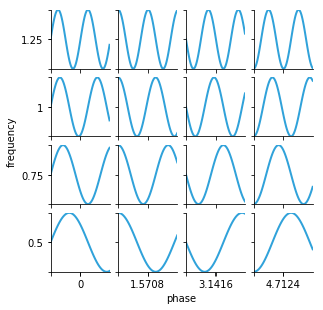Gridspace¶

Title
GridSpace Container
Dependencies
Matplotlib
Backends
Matplotlib
Bokeh
Plotly
In :
import numpy as np
import holoviews as hv
hv.extension('matplotlib')A GridSpace is a two-dimensional dictionary of HoloViews objects presented onscreen as a grid. In one sense, due to the restriction on it's dimensionality, a GridSpace may be considered a special-case of HoloMap. In another sense, GridSpace may be seen as more general as a GridSpace can hold a HoloMap but the converse is not permitted; see the Building Composite Objects user guide for details on how to compose containers.

GridSpace holds two-dimensional dictionaries¶

Using the sine_curve function below, we can declare a two-dimensional dictionary of Curve elements, where the keys are 2-tuples corresponding to (phase, frequency) values:

In :
def sine_curve(phase, freq):
xvals = [0.1* i for i in range(100)]
return hv.Curve((xvals, [np.sin(phase+freq*x) for x in xvals]))

phases      = [0, np.pi/2, np.pi, 3*np.pi/2]
frequencies = [0.5, 0.75, 1.0, 1.25]
curve_dict_2D = {(p,f):sine_curve(p,f) for p in phases for f in frequencies}

We can now pass this dictionary of curves to GridSpace:

In :
gridspace = hv.GridSpace(curve_dict_2D, kdims=['phase', 'frequency'])
gridspace
Out:GridSpace is similar to HoloMap¶

Other than the difference in the visual semantics, whereby GridSpaces display their contents together in a two-dimensional grid, GridSpaces are very similar to HoloMaps (see the HoloMap notebook for more information).

One way to demonstrate the similarity of these two containers is to cast our gridspace object to HoloMap and back to a GridSpace:

In :
hmap = hv.HoloMap(gridspace)
hmap + hv.GridSpace(hmap)
Out: